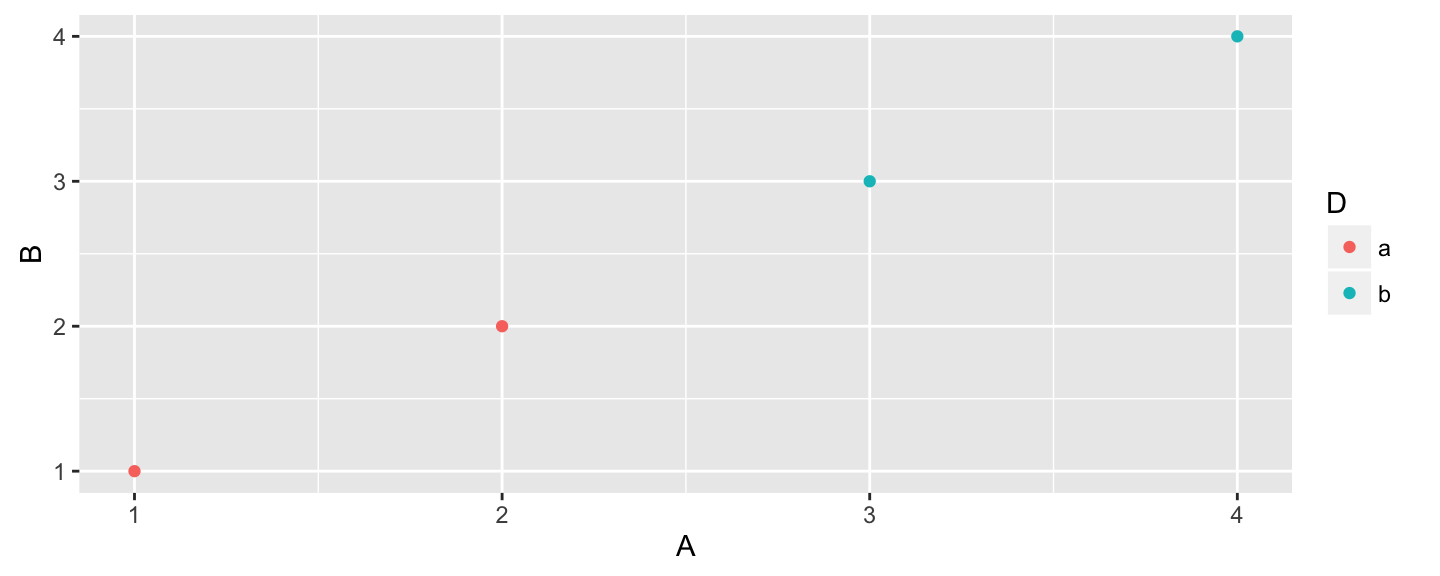Mon Sep 26, 2016

## 5NG

Today:

1. Scatterplot AKA bivariate plot
2. Line-graph
3. Boxplot
4. Barplot AKA Barchart AKA bargraph
5. Histogram

## Example

Let's re-run our example from last time:

# Load packages
library(dplyr)
library(ggplot2)

# Create data frame
simple_ex <-
data_frame(
A = c(1, 2, 3, 4),
B = c(1, 2, 3, 4),
C = c(3, 2, 1, 2),
D = c("a", "a", "b", "b")
)

simple_ex

## Example

A statistical graphic is a mapping of data variables to aes()thetic attributes of geom_etric objects.## Today

• Drill down on geom_point()
• Introduce two techniques for over-plotting. See saw this phenomenon in the Lec05 Learning Check discussion.
• alpha to control transparency
• geom_jitter(): a kind of geom_point() where we add a little jitter (i.e. random noise) to the points to break log-jams